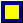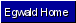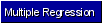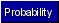Egwald Statistics: Probability and Stochastic Processes by Elmer G. Wiens Egwald's popular web pages are provided without cost to users. Please show your support by joining Egwald Web Services as a Facebook Fan:Follow Elmer Wiens on Twitter:Probability Distributions. A. Bivariate Normal Distribution: Given the random variable X1, with mean u1and standard deviation s1, and random variable X2, with mean u2 and standard deviation s2. Let the correlation coefficient of X1 and X2 be rho. The bivariate normal density is defined as: f(x1, x2) = exp[(-0.5/(1-rho^2)) * ((x1 - u1)/ s1 )^2) - 2*rho*((x1 - u1)/ s1)*((x2 - u2)/ s2) + ((x2 - u2)/ s2 )^2)]  / [2* pi * s1 * s2 * sqrt(1-rho^2)] where u1 and s1 are the mean and standard deviation of the marginal distribution of x1: f1(x1) = exp[-0.5) * ((x1 - u1)/ s1 )^2)]/ [sqrt(2* pi) * s1] and, where u2 and s2 are the mean and standard deviation of the marginal distribution of x2: f2(x2) = exp[-0.5) * ((x2 - u2)/ s2 )^2)]/ [sqrt(2* pi) * s2] Bivariate Normal Density with u1 = 0, s1 = 1, u2 = 0, s2 = 1, rho = .7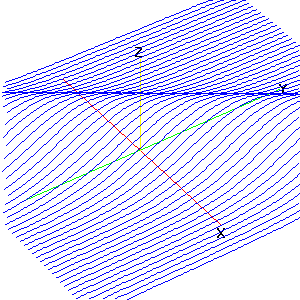B. Conditional Bivariate Normal Distribution: The conditional density of x1 given x2 f(x1 | x2) = f(x1, x2) / f2(x2) f(x1 | x2) = exp[(-0.5/(1-rho^2)) * {(1/s1)*(x1 - (u1 + rho*(s1/s2)*(x2 - u2))}^2] / [sqrt(2* pi) * s1 * sqrt(1-rho^2)] The mean of f(x1 | x2) is   (u1 + rho*(s1/s2)*(x2 - u2)) while the standard deviation f(x1 | x2) is   s1*sqrt(1-rho^2). As x2 increases, the graph of f(x1 | x2) shifts to the right. Conditional Bivariate Normal Density with u1 = 5, s1 = 1.5, u2 = 10, s2 = 2, rho = .3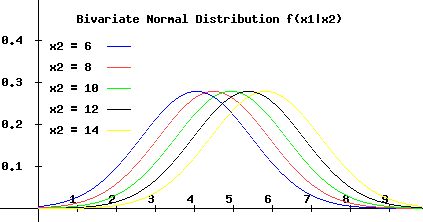As rho increases, from 0 to 1, the graph of f(x1 | x2) increases in height, and its width decreases about the mean of   f(x1 | x2). Conditional Bivariate Normal Density with u1 = 5, s1 = 1.5, u2 = 10, s2 = 2, rho = .7Copyright © Elmer G. Wiens:   Egwald Web ServicesAll Rights Reserved.    Inquiries